# 4 4 STANDARD DEVIATION STANDARD DEVIATION The Standard

• Slides: 11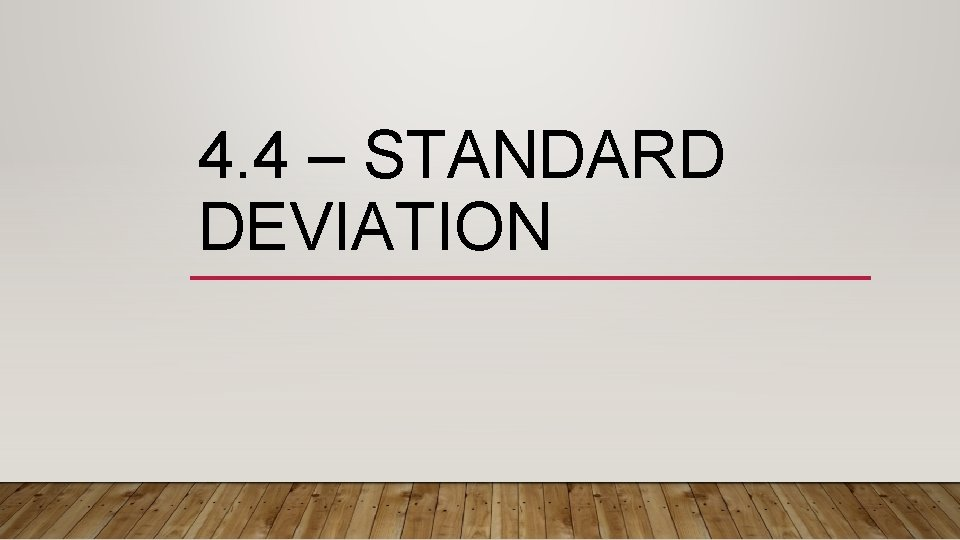4. 4 – STANDARD DEVIATIONSTANDARD DEVIATION • The Standard Deviation is a measure of how spread out numbers are. • σ (the greek letter sigma) • In order to calculate the standard deviation we must first find the variance of data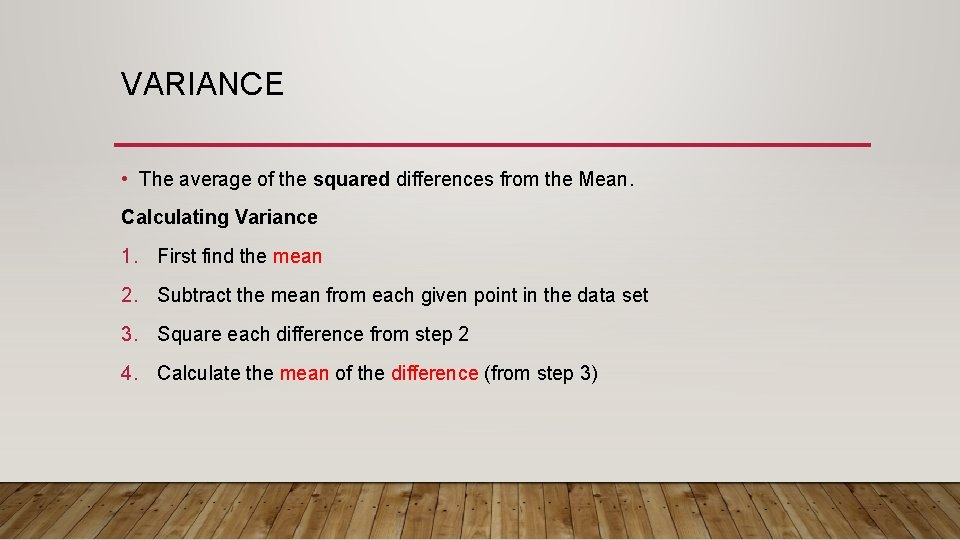VARIANCE • The average of the squared differences from the Mean. Calculating Variance 1. First find the mean 2. Subtract the mean from each given point in the data set 3. Square each difference from step 2 4. Calculate the mean of the difference (from step 3)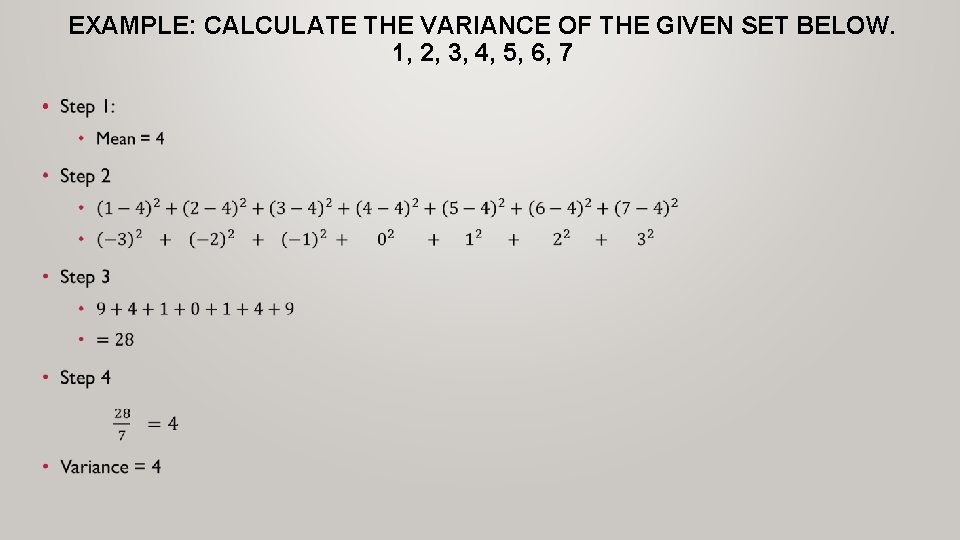EXAMPLE: CALCULATE THE VARIANCE OF THE GIVEN SET BELOW. 1, 2, 3, 4, 5, 6, 7 •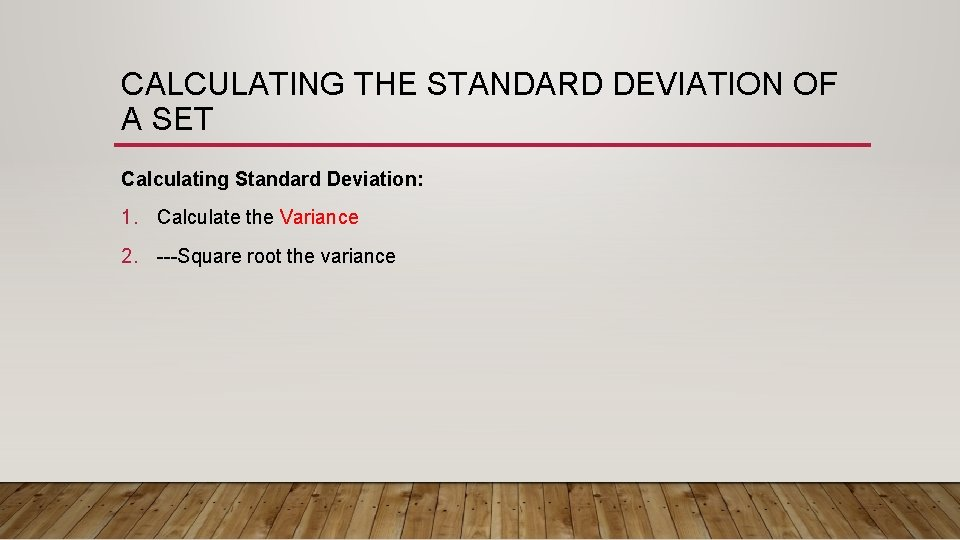CALCULATING THE STANDARD DEVIATION OF A SET Calculating Standard Deviation: 1. Calculate the Variance 2. Square root the variance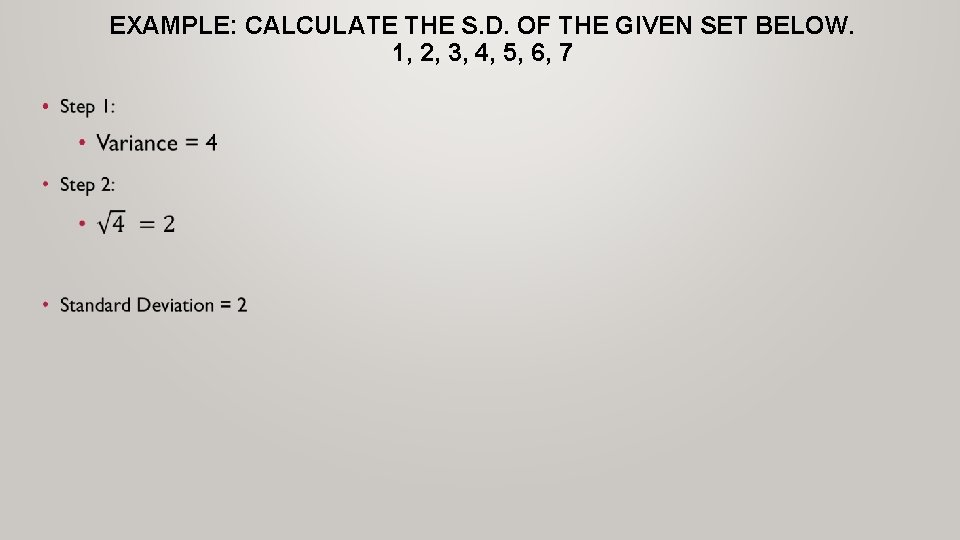EXAMPLE: CALCULATE THE S. D. OF THE GIVEN SET BELOW. 1, 2, 3, 4, 5, 6, 7 •PERCENTAGES OF NORMAL DISTRIBUTIONS • 68. 2% of all data that is normally distributed should be within one standard deviation. • 95. 5% of all data that is normally distributed should be within two standard deviation. • 99. 7% of all data that is normally distributed should be within three standard deviation.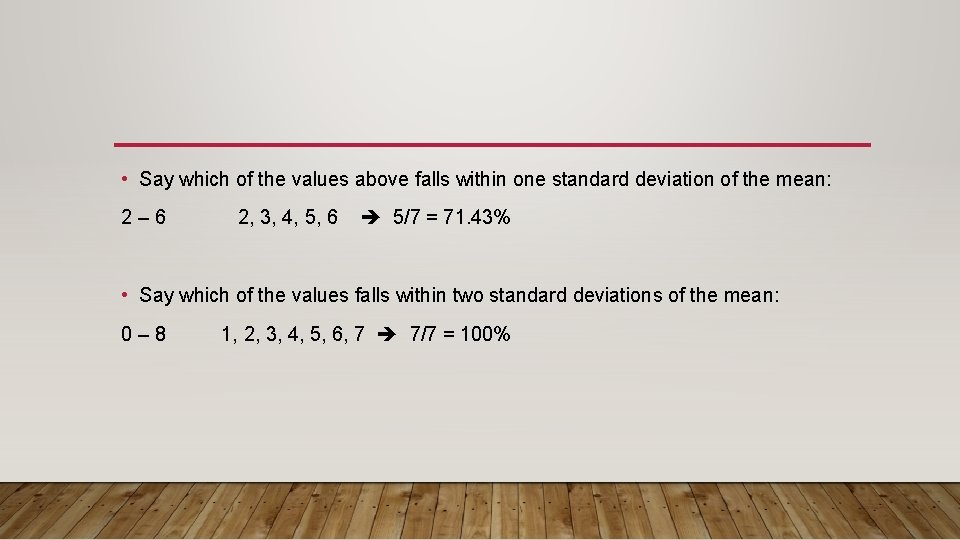• Say which of the values above falls within one standard deviation of the mean: 2 – 6 2, 3, 4, 5, 6 5/7 = 71. 43% • Say which of the values falls within two standard deviations of the mean: 0 – 8 1, 2, 3, 4, 5, 6, 7 7/7 = 100%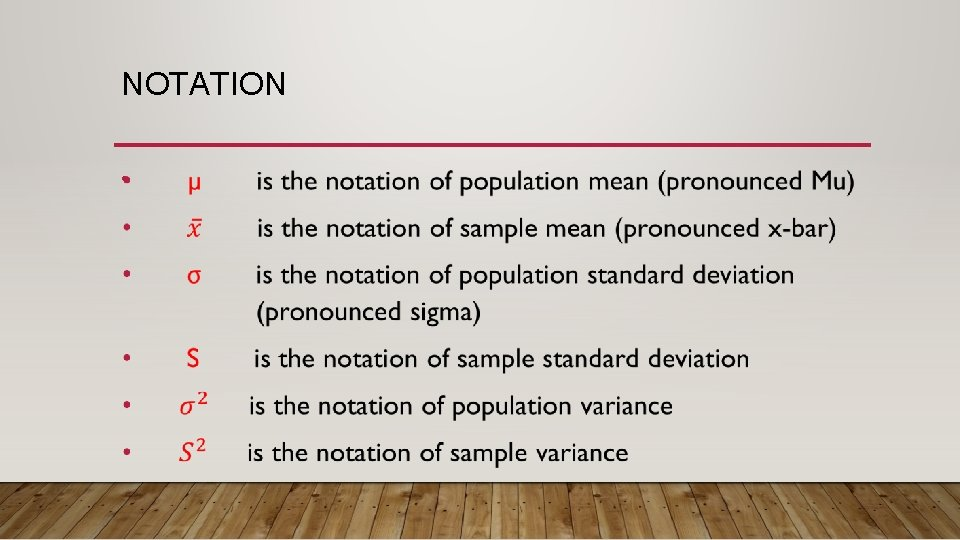NOTATION •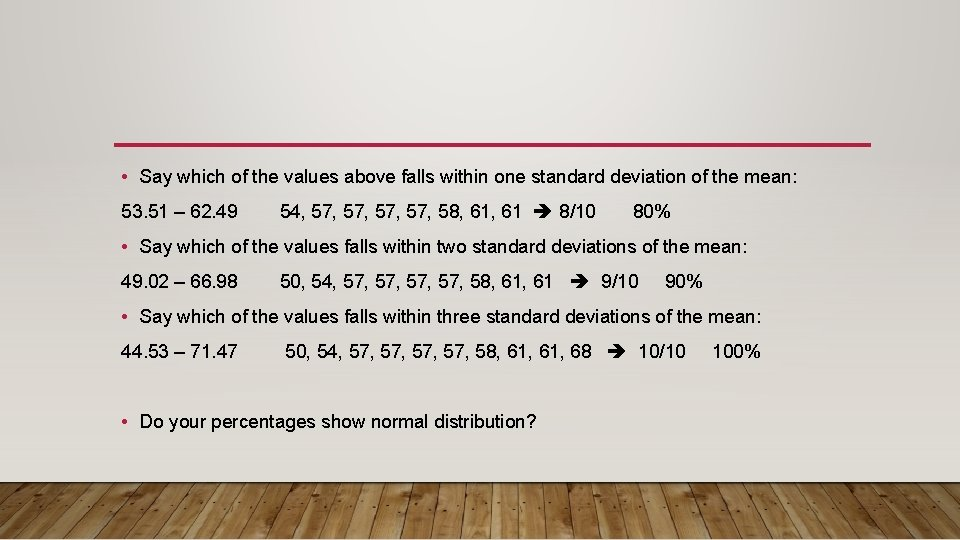• Say which of the values above falls within one standard deviation of the mean: 53. 51 – 62. 49 54, 57, 57, 58, 61 8/10 80% • Say which of the values falls within two standard deviations of the mean: 49. 02 – 66. 98 50, 54, 57, 57, 58, 61 9/10 90% • Say which of the values falls within three standard deviations of the mean: 44. 53 – 71. 47 50, 54, 57, 57, 58, 61, 68 10/10 100% • Do your percentages show normal distribution?